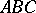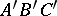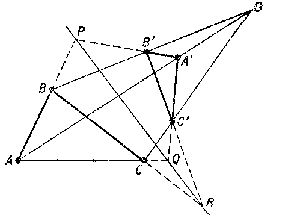# Desargues assumption

(diff) ← Older revision | Latest revision (diff) | Newer revision → (diff)

Desargues theorem

If the corresponding sides of two trianglesandintersect at pointson the same straight line, then the straight lines which connect the corresponding vertices intersect at one point. Conversely, if the straight lines connecting the corresponding vertices of the trianglesandintersect at one point, the corresponding sides of these triangles intersect at points lying on a straight line. The converse statement for triangles lying in the same plane is dual to the statement for straight lines by the little duality principle. In both cases the points and the straight lines constitute a Desargues configuration (a-configuration), located in some two-dimensional or three-dimensional projective space.Figure: d031320a

If both triangles belong to the same projective space, the Desargues assumption cannot be demonstrated on the strength of plane incidence axioms alone (cf. Non-Desarguesian geometry), but it is valid for any projective plane which may be imbedded in a projective space of larger dimension. The spatial case of the Desargues assumption follows from the space incidence axioms.

The validity of the Desargues assumption is necessary and sufficient for the construction of the projective algebra of points of the projective straight line and for the synthetic introduction of projective coordinates. Established by G. Desargues, .

## Contents

How to Cite This Entry:
Desargues assumption. Encyclopedia of Mathematics. URL: http://encyclopediaofmath.org/index.php?title=Desargues_assumption&oldid=14646
This article was adapted from an original article by M.I. Voitsekhovskii (originator), which appeared in Encyclopedia of Mathematics - ISBN 1402006098. See original article